# HBSE 10th Class Maths Notes Chapter 13 Surface Areas and Volumes

Haryana State Board HBSE 10th Class Maths Notes Chapter 13 Surface Areas and Volumes Notes.

## Haryana Board 10th Class Maths Notes Chapter 13 Surface Areas and Volumes

Introduction
In previous classes, we have learnt about surface areas and volumes of solids like cuboid, right circular cylinder, right circular cone and sphere. In this chapter we shall use our previous knowldege about these solids and learn about such solids which are combinations of two or more solids. For example a test tube which is combination of a cylinder and a hemisphere, an ice cream cone is a combination of a cone and a hemishpere, conical circus tent with cylindrical base is combination of a right circular cylinder and right circular cone etc. We shall find the surface area and volume of such solids.

In this chapter, we shall also discuss problems on surface area and volume of frustum of a cone for example bucket, glass tumbler, a friction clutch etc.

Some important terms are following:
1. Solids: Bodies which have three dimensions in space are called solids.
2. Volume: The amount of space occupied by a solid or bounded by a closed surface is known as the volume of solid.
3. Surface area: Surface area is the total sum of all the areas of all the shapes that cover the surface of solid.
4. Lateral surface area: Lateral surface in a solid is the sum of surface areas of all its faces excluding the bases of solid.
5. Cuboid: A cuboid is a solid bounded by six rectangular plane regions.
6. Cube: When all the edges of cuboid are equal in length, it is called a cube.
7. Right circular cylinder: If a rectangle is revolved about one of its sides, the solid thus formed is called right circular cylinder.
8. Right circular cone: If a right angled triangle is revolved about one of the sides containing a right angle, the solid then generated is called a right circular cone.
9. Sphere: The set of all points in space which are equidistant from a fixed point, is called a sphere.
10. Hemisphere: A plane through the centre of a sphere divides the sphere into equal parts, Each part is called a hemisphere.
11. Spherical shell: A spherical shell is the difference between the two solid concentric spheres.
12. Slant height: The slant height of a right circular cone is the distance measured along the lateral surfaces from any point on the circular base to top apex of the cone.
13. Frustum of a right circular cone: If a right circular cone is cut off by a plane parallel to its base. The portion of the cone between the plane and base of the cone is called a frustum of the cone. The shape of this part (Frustum of cone is like that of a bucket, funnels cans, etc.)Surface area of a Combination of Solids Cuboid
If l, b and h denote respectively the length, breadth and height of a cuboid.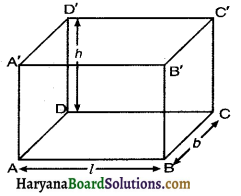Then
(i) Volume of cuboid = l × b × h cubic units
= Area of the base × height
(ii) Total surface area of a cuboid = 2(lb + bh + hl) square units
(iii) Surface area of 4 walls of a room = 2(l + b) × h square units
(iv) Surface area of cuboid, in which top face is open = lb + 2(bh + hl) square units
(v) Diagonal of cuboid = $$\sqrt{l^2+b^2+h^2}$$ units

Cube:
If all lengths of the edges of a cuboid are equal, then it is called a cube.
Let the length of each edge of cube be “a” units.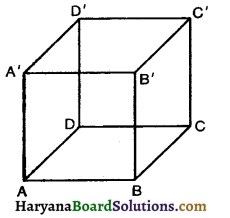(i) Volume of cube = a3 cubic units
(ii) Total surface area of cube = 6a2 square units
(iii) Diagonal of cube = $$\sqrt{3}$$a units
(iv) Edge of cube = $$\sqrt{\text { Volume }}$$

Right circular cylinder:
If radius of base is r and height h, then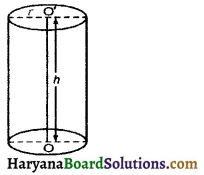(i) Volume of cylinder = Area of base × Height
= πr2h cubic units
(ii) Curved surface area or lateral surface area of cylinder
= Perimeter of base × Height
= 2πrh square units
(iii) Total surface area of cylinder = Curved surface area + Area of two circular ends
= 2πrh + 2πr2
= 2πr (h + r) square units

Right circular hollow cylinder:
If R and rare external and internal radii of a right circular hollow cylinder of height h. Then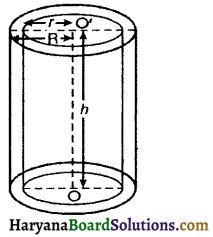(i) Area of each end = π (R2 – r2)
(ii) Volume of material = Exterior volume – Interior volume
= πR2h – πr2h
= πh (R2 – r2) cubic unita
(iii) Curved surface area = External surface area + Internal surface area
= 2πRh + 2πrh
= 2πh (R + r) square units
(iv) Total surface area = curved surface area + Area of base rings
= 2πRh + 2πrh + 2π (R2 – r2)
= 2πh(R + r) + 2π(R + r) (R – r)
= 2π (R + r) (h + R – r) square unitsRight circular cone:
Consider a cone in which Radius of base = r, Height = h, and slant height (l) = $$\sqrt{h^2+r^2}$$. Then,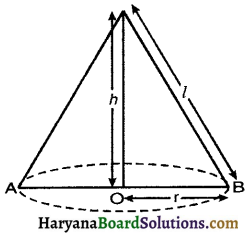(i) Volume of the cone = $$\frac{1}{3}$$πr2h cubic units
= $$\frac{1}{3}$$(Area of buse) × height
(ii) Curved surface area of cone = πrl sq. units
(iii) Total surface of the cone = Curved surface + Area of base
= πrl + πr2
= πr(l + r) sq. units

Sphere:
For a sphere of radius r. we have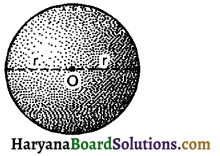(i) Volume of sphere = $$\frac{4}{3}$$πr3 cubic units
(ii) Surface area of sphere = 4πr2 square units

Hemisphere:
For a hemishpere of radius r.
We have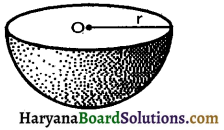(i) Volume of hemisphere = $$\frac{2}{3}$$πr3 cubic units
(ii) Curved surface area of hemisphere = 2πr2 sq. unita
(iii) Total surface Area of hemisphere = 3πr2 sq. units
In our daily life, we come across different solids which are combinations of different solids (cube, right circular cylinder, right circular cone, sphere, hemisphere, etc.).

For example: An ice-cream cone consisting a cone and a hemisphere, a circus tent which is combination of right circular cylinder and a right circular cone. A capsule is the form of a cylinder with hemispherical ends etc. Following examples will illustrate the method of finding surface areas and volumes of such combinations of solids.Volume of a combination of solids
In the previous section, we have discussed how to find the surface area of solids made up of a combination of two basic solids. In this section, we shall explain the method of finding volumes of solids which are made by the combination of basic solids. The volume of the solid formed by joining two basic solids will actually be the sum of the volumes of the constituents, as we see in examples given below.

Conversion of solid from one shape to another
At times we may convert objects from one shape to another objects with different shapes or when a liquid which originally filled one container of a particular shape is poured into another container of a different shape or size.

For examples: A metallic sphere melted and recast into smaller cones. Spherical bullets made out of the solid cube. A sphere may be melted and recast into a wire, etc. For solving such types of problems the surface area will be change only and no change of volume takes place. Let us consider some examples to clear the method of conversion of solids from one shape to another.

Frustum of a right circular cone
If a right circular cone is cut off by a plane parallel to its base, the portion of the cone between the plane and base of the cone is called a frustum the cone. The shape of this part (Frustum of cone is like that of a bucket, funnels, flower pots, cans, jugs, lampshades etc.)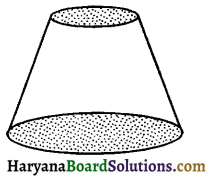We consider a cone (VAB) which cuts by a plane CD parallel to the base AB through some point (0) on its axis and the portion containing the vertex (V) is removed. The left portion ACDB is usually called frustum of the cone.
A frustum of a right circular cone has two unequal flat circular bases and a curved surface.

The line segment OP joining the centres of two bases called the height of the frustum. Each of line segment AC and BD of frustum ACDB is called its slant height.Volume and surface area of frustum
Let r1 and r2 be the radii of the circular end bases (r1 > r2) of the frustum ABCD of the cone (VAB). Let h and l be the vertical height and slant height respectively. Then OP = h and AC = BD = l. The frustum of right circular cone can be viewed as the difference of the two right circular cone VAB and VCD. Let the height of cone VAB be h1 and its slant height be l1, i.e, VP = h1 and VA = VB = l1
Height of the cone (VCD) = VP – OP = h1 – h
Right ΔVOD ~ right ΔVPB (By AA similarity)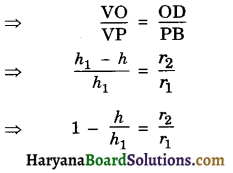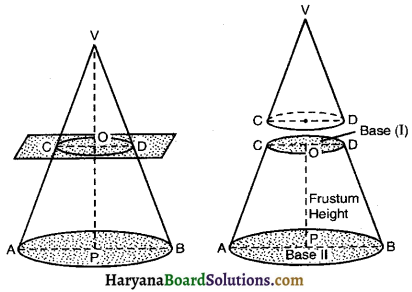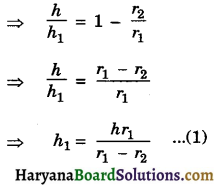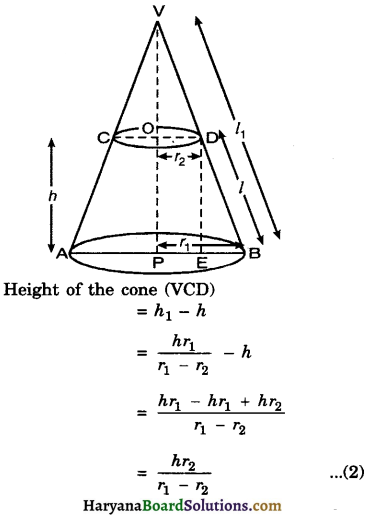Volume of the frustum ACDB of the cone (VAB)
= Volume of cone (VAB) – Volume of the cone (VCD)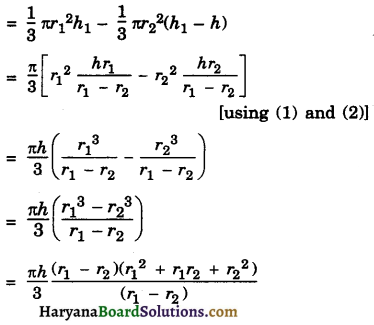$$\frac{1}{3}$$πh(r12 + r1r2 + r22)
Hence, volume of the frustum of cone = $$\frac{1}{3}$$πh(r12 + r1r2 + r22).
Again if A1 and A2 (A1 > A2) are the surface areas of the two circular bases. Then
A1 = πr12 and A2 = πr2
Volume of the frustum of cone
= $$\frac{1}{3}$$πh(r12 + r22 + r1r2)
= $$\frac{h}{3}$$ (πr12 + πr22 + $$\left.\sqrt{\pi r_1^2} \times \sqrt{\pi r_2^2}\right)$$}
Hence, volume of frustum of cone
= $$\frac{h}{3}$$(A1 + A2 + $$\sqrt{\mathrm{A}_1 \mathrm{~A}_2}$$)
Now form right ΔDEB,
DB2 = DE2 + BE2.
l2 = h2 + (r1 – r2)
[where BE = r1 – r2]
⇒ l = $$\sqrt{h^2+\left(r_1-r_2\right)^2}$$
Again ΔVOD ~ ΔVPB (By AA similarity of criterion)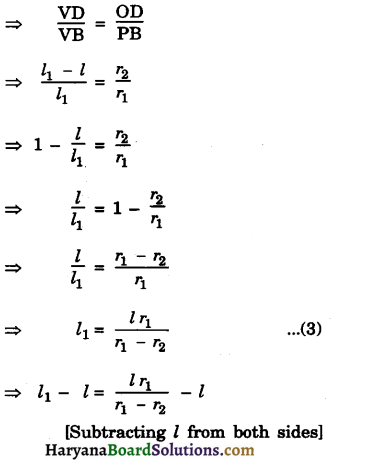⇒ $$l_1-l=\frac{l r_1-l r_1+l r_2}{r_1-r_2}$$
⇒ $$l_1-l=\frac{l r_2}{r_1-r_2}$$ ……..(4)
Curved surface of the furstum of cone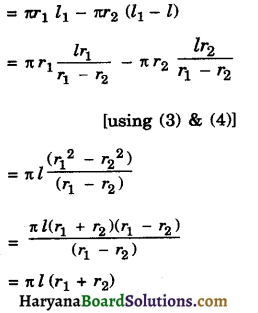Hence, curved surface of the frustum of right circular cone
= πl(r1 + r2)
where l = $$\sqrt{h^2+\left(r_1-r_2\right)^2}$$
Total surface area of frustum of right circular cone = curved surface area + area of base 1 + area of base 2
= πl(r1 + r2) + πr12+ πr22
= π[r12 + r22 + l (r1 + r1)]
Hence, total surface area of frustum of right circular cone = π[r12 + r22 + l(r1 + r2)].